吴恩达机器学习笔记-应用机器学习的建议

评估假设

• 获取更多的训练集
• 减少特征的数量
• 尝试使用更多的特征
• 尝试使用多项式特征
• 增大或减小lambda

J_{test}(\Theta) = \frac{1}{2m_{test}}\sum_{i=1}^{m_{test}}(h_\Theta(x_{test}^(i)))^2

h_\Theta(x)>=0.5且y=0h_\Theta(x)<0.5且y=1 时,err(h_\Theta(x),y) = 1;否则，err(h_\Theta(x),y) = 0

Test Error = \frac{1}{2m_{test}}\sum_{i=1}^{m_{test}}err(h_\Theta(x_{test}^(i)),y_{test}^(i))

模型选择-训练集、验证集、测试集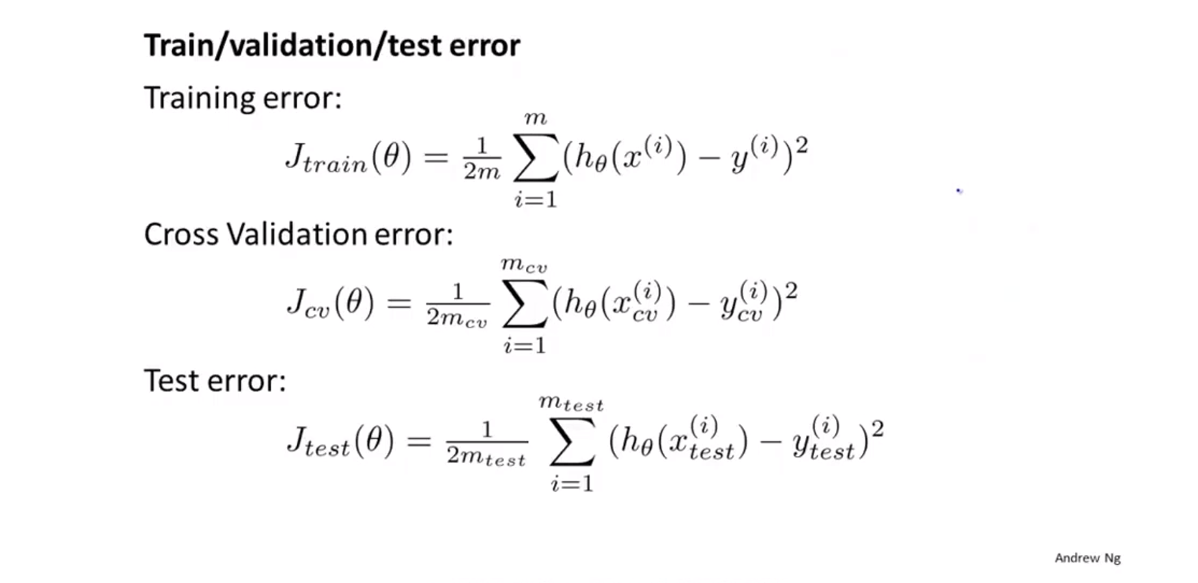1. 通过训练集来针对每一个级数的多项式来最优化参数theta
2. 通过交叉验证集来找到最误差最小的级数d
3. 使用J_{test}(\Theta^d)来估计使用测试集得到的模型的泛化误差，这样的话，多项式的级数就不用通过测试集训练得到了。

诊断偏差与方差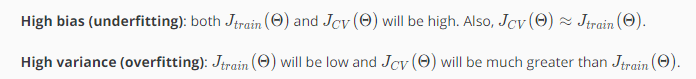正则化和偏差、方差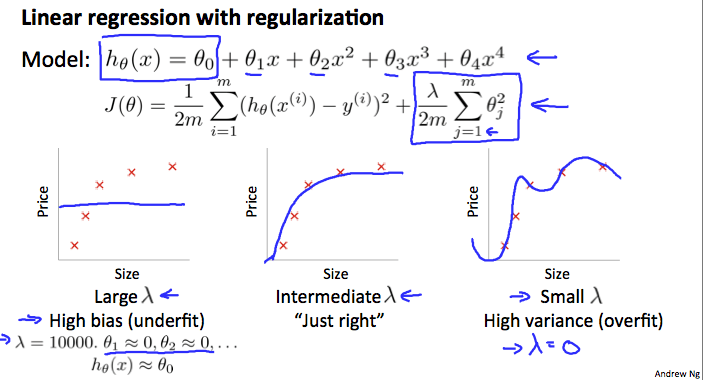1. Create a list of lambdas (i.e. λ∈{0,0.01,0.02,0.04,0.08,0.16,0.32,0.64,1.28,2.56,5.12,10.24});
2. Create a set of models with different degrees or any other variants.
3. Iterate through the λs and for each λ go through all the models to learn some \ThetaΘ.
4. Compute the cross validation error using the learned Θ (computed with λ) on the J_CV ​(Θ)  without regularization or λ = 0.
5. Select the best combo that produces the lowest error on the cross validation set.

Using the best combo Θ and λ, apply it on J_test ​(Θ)  to see if it has a good generalization of the problem.

\lambda的取值和J(\theta)的曲线画出来如下所示：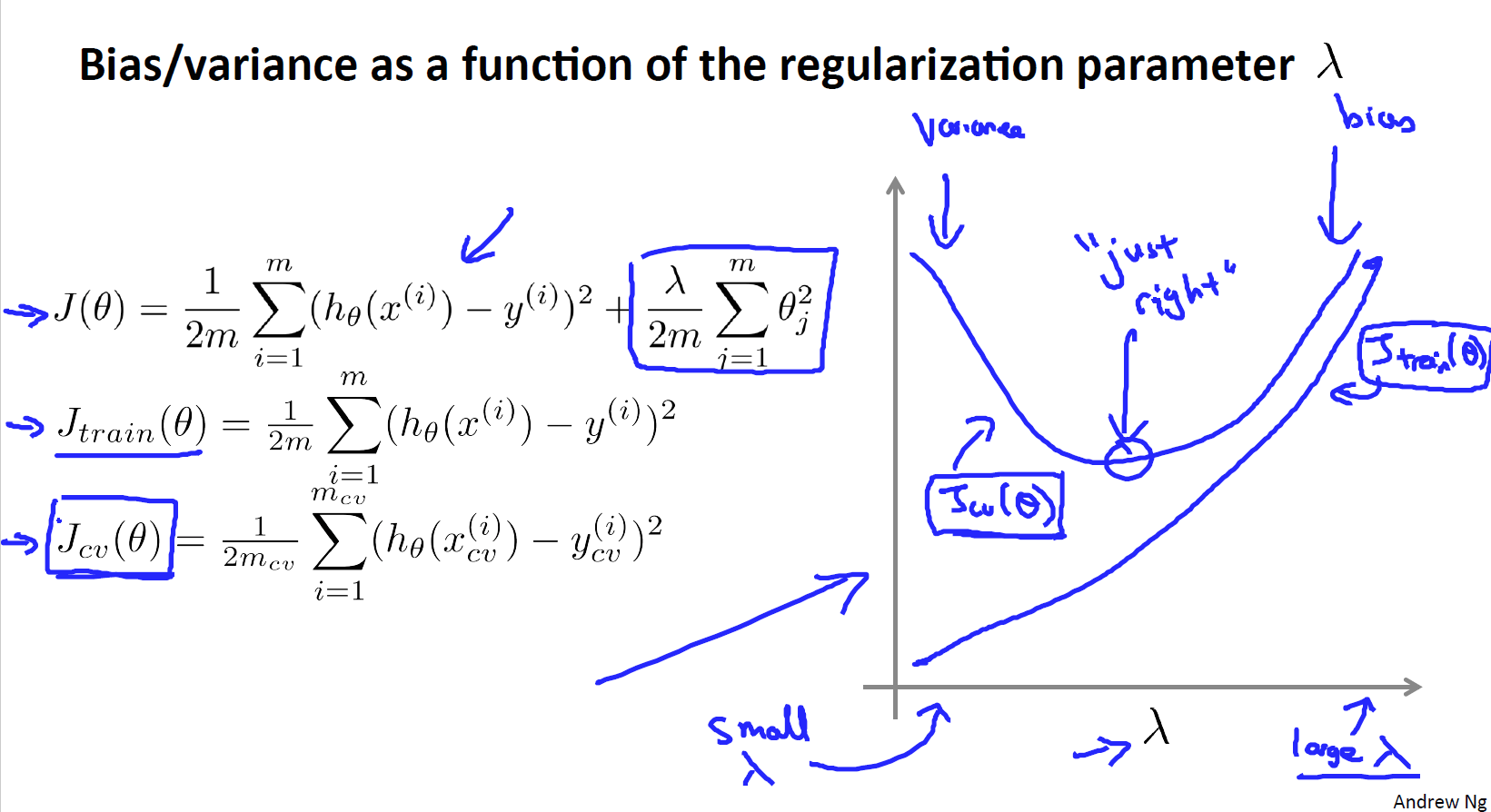J_{train}(\theta)随着\lambda值的增加而增加，因为\lambda的值增大会对应着高偏差问题，此时连训练集都不能很好的拟合，当\lambda很小时，对应着你可以很容易地用高次多项式拟合你的数据。对于交叉验证的误差，如图中粉色的图像所示，当\lambda很大时，会出现欠拟合问题，因此是那一片是高偏差区域，此时交叉验证集的误差会很大，当\lambda很小时，会出现过拟合问题，对应的是高方差问题，因此交叉验证集的误差也会很大。同样的，总会有中间的某个点对应的\lambda表现的结果正好合适，交叉验证误差和测试误差都很小。

学习曲线

• 当训练集增大时，二次函数的误差将会增加
• 随着集合数据的增加，最终的误差会渐渐趋向于平稳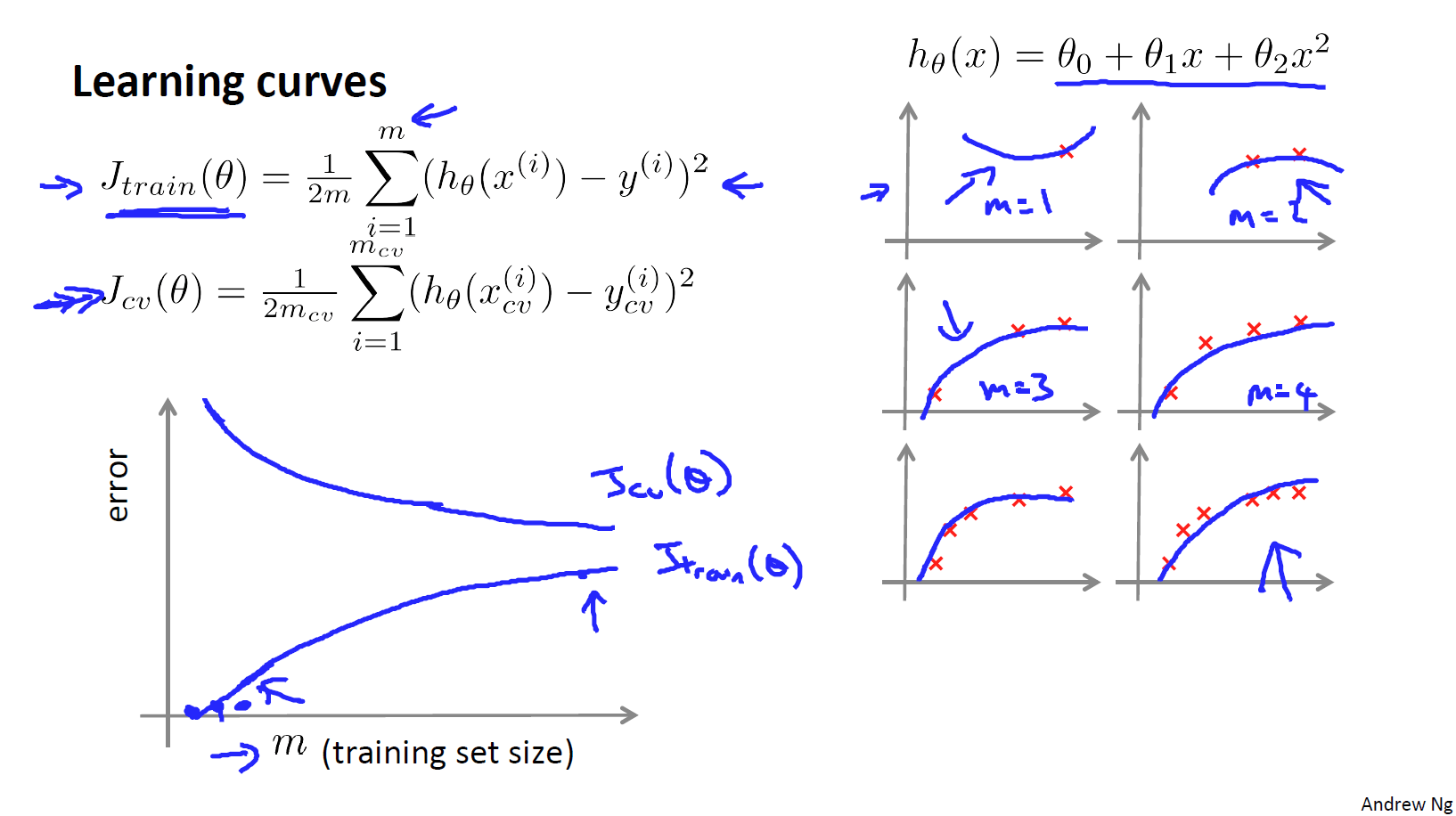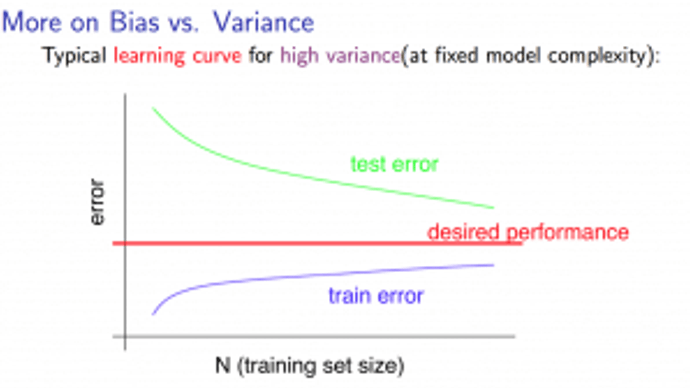总结

• 获取更多的训练集 --> 解决高方差问题
• 减少特征的数量 --> 解决高方差问题
• 尝试使用更多的特征 --> 解决高偏差问题
• 尝试使用多项式特征 --> 解决高偏差问题
• 增大lambda --> 解决高方差问题
• 减小lambda --> 解决高偏差问题

• 一个相对简单的神经网络模型，隐含单元比较少或者隐藏层很少时，那么拟合时选择的参数就会比较小，容易出现欠拟合，但他的优势
• 拟合一个复杂的神经网络模型时，那么其参数一般比较多，容易出现过拟合，这种结构的计算量相对会很大。但过拟合问题可以使用正则化来进行修正。通常使用大型网络模型然后使用正则化来防止过拟合问题相对于使用简单的神经网络拟合的效果要更好。

• 低阶多项式，也就是低复杂程度的模型有高偏差和低方差的问题，这种情况下，模型的拟合程度较差
• 高阶多项式，也就是高复杂程度的模型对于训练集的拟合效果相对较好，但对于测试集的拟合效果非常差，会出现低偏差和高方差问题。
• 在现实中，我们需要在两者之间选择一个合适的模型，使其能很好的来拟合数据。